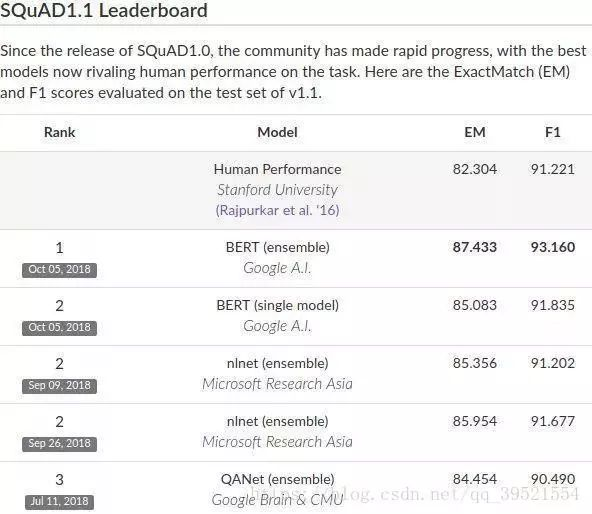Transformer是谷歌发表的论文《Attention Is All You Need》提出一种完全基于Attention的翻译架构

### VIP内容080

### 热门内容BERT好文分享

•0391

### 最新内容000

### 最新论文We provide a graphical treatment of SAT and \#SAT on equal footing. Instances of \#SAT can be represented as tensor networks in a standard way. These tensor networks are interpreted by diagrams of the ZH-calculus: a system to reason about tensors over $\mathbb{C}$ in terms of diagrams built from simple generators, in which computation may be carried out by \emph{transformations of diagrams alone}. In general, nodes of ZH diagrams take parameters over $\mathbb{C}$ which determine the tensor coefficients; for the standard representation of \#SAT instances, the coefficients take the value $0$ or $1$. Then, by choosing the coefficients of a diagram to range over $\mathbb B$, we represent the corresponding instance of SAT. Thus, by interpreting a diagram either over the boolean semiring or the complex numbers, we instantiate either the \emph{decision} or \emph{counting} version of the problem. We find that for classes known to be in P, such as $2$SAT and \#XORSAT, the existence of appropriate rewrite rules allows for efficient simplification of the diagram, producing the solution in polynomial time. In contrast, for classes known to be NP-complete, such as $3$SAT, or \#P-complete, such as \#$2$SAT, the corresponding rewrite rules introduce hyperedges to the diagrams, in numbers which are not easily bounded above by a polynomial. This diagrammatic approach unifies the diagnosis of the complexity of CSPs and \#CSPs and shows promise in aiding tensor network contraction-based algorithms.000下载预览
Top